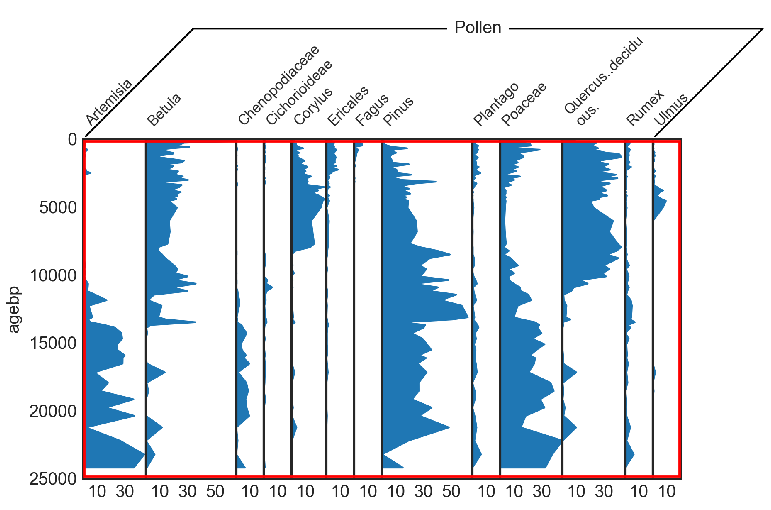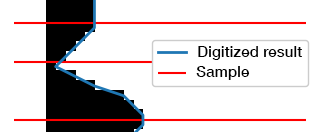# Basics and Terminology¶A stratigraphic diagram consists of multiple diagrams that are vertically or horizontally aligned, i.e. that have either the same x- or y-axis.

This software assumes, that all the subdiagrams share the y-axis, i.e. the vertical axis. If this is not the case for your specific diagram, just rotate your image by 90°.

column

One column in the diagram means one of the subdiagrams (e.g. Betula in the example diagram above). The total number of columns is the number of subdiagrams in your plot.

pixel column

One pixel column is the horizontal pixel index (x-axis), ranging from 0 to the width of your image. Pixel column 0 represents all pixels on the very left side.

row or pixel row

One pixel row (or sometimes only row) is the vertical pixel index (y-axis), ranging from 0 to the height of your image. Row 0 represents all pixels at the top.

diagram part

This is the part where the data is shown (red rectangle in the figure above).

binary image

The binary image is the diagram part converted to black (1) and white (0). Every black pixel in this binary image represents data that should be digitized.

digitize

Digitization means transforming each pixel into a value (see the blue line in the image above). Therefore, in contrast to the samples, you get one data value for each pixel row in the binary image. We also refer to this as the full data.sample

The samples (red lines in the image above) are a subset of the digitization result. In a very basic sense, the samples represent the original data that was used to create the diagram. In a sediment core for a pollen diagram, it would be one slice of the core that was analyzed under the microscope.

Everything that refers to the straditizer refers to the full image of your stratigraphic diagram. Speaking in a programmatically sense, it is the top-level interface to digitize the diagram, an instance of the `straditize.straditizer.Straditizer` class. You can always access the current straditizer through the `stradi` variable in the ipython console of the GUI
The reader of your diagram can be accessed via typing `stradi.data_reader` in the ipython console of the GUI.